### Home > PC > Chapter 12 > Lesson 12.2.1 > Problem12-42

12-42.
1. Recall from Chapter 1 that the line through the points A and B can be described by the vector equation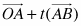for −∞ < t < ∞. Homework Help ✎

1. Let A = (2, 4) and B = (1, −3). Since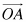= 2i + 4j and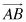= −i − 7j, verify that in this case+ t() = 2i + 4j + t(−i − 7j).

2. Rewrite this expression as ( )i + ( )j.

3. Show that this line goes through the point (0, −10).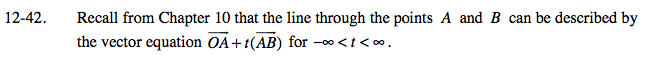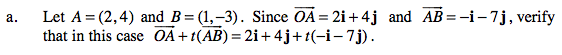Use substitution.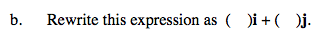Use the distributive property, then combine like terms.

2i + 4j + t(−i −7j)
= 2i + 4jti −7tj
= 2iti + 4j −7tj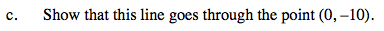If (0, 10) is on the line, then 0i + 10j is possible.

(2 − t)i + (4 − 7t)j = 0i + 10j

2 − t = 0 4 − 7t = 10

Does t have the same value in both equations?
If it is on the line, then it should.